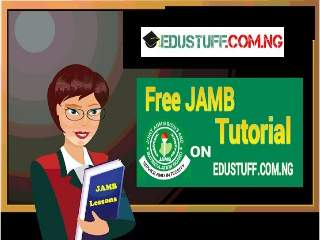# JAMB Free Tutorial 2022: WORD PROBLEM SECRETS REVEALED (PART 1️⃣)

4

JAMB Free Tutorial : WORD PROBLEM SECRETS REVEALED(PART 1️⃣)

We have received several requests from students, telling us to start a tutorial, we’ve been thinking on how to set it up since then and this is for Mathematics.

Every time while tutoring students in mathematics, I have discovered most  students are weak in one major topic  “Word problems”.As this topic requires understanding of letters and figures and how to manipulate them.
We at Edustuff want to eradicate the fears of students on this topic with simple questions.

### What is Word Problem?

Word problem by definition are mathematical problems which are written in word.
Which means to solve word problem, you must understand some important words used.

Some of them are :

• Sum: Sum Is the result we get when we add two or more numbers together e.g  The sum of 20 and 17 is 37.

==========
The sum of 5,10 and 6 is 21
==========
The sum of 3 and a number is?
This is a basic problem.
We first assume the number to be  Letter “X
So the sum of 3 and a number will be  X + 3.
=======
The sum of 5 and a number is equal to 25,find the number?
Difficult?
No it shouldn’t be!!
Let the unknown number be  x
So the sum of 5 and a number =25
X +5=25
X=25 – 5
X = 20
Check : 20 + 5 =25.
Easy??
Let’s move on

•  Difference : the result of subtracting one number from the other. It can be positive or negative

======≠=≠====

1. Positive difference : The positive difference between 24 and 10 is 14

It means the bigger number – smaller number
=============

2.Negative difference  : The negative difference between  2 and 5 means the smaller – bigger.
2 – 5 = -3

• PRODUCT : This is the result of multiplying two or more numbers

E.g : The product of 2 and 9 is?
Solution
=========
2 ✖ 9  = 18
===========
The product of  3 and a number gives 18,Find the number?
Simple right?
Let the number be  x or any arbitrary alphabet  as usual.. Let’s say A for this question
Product means multiplication
3 multiplied by a number =18
3 ✖  A =18
3A = 18
Divide both side by 3
3A =18
—– ——
3      3
A= 6
Check : 3✖ 6 = 18
==============
3 multiplied by the sum of 2 and a number gives 12. Find the number
Hard right.?
U have to stay calm and break down the questions
============
3 multiplied by the sum (X+2) gives 12

3(X+2) =12
Open the bracket by multiplying by 3

3X + 6 = 12
3X =12 – 6
3X = 6
Divide both side by 3
3X =6
—-  ——
3     3
X = 2
Check :
3(2+2)= 12
3 ✖4=12
12=12
Correct!!!!

•  Consecutive odds numbers : odd numbers that follow themselves in array e.g “1,3,5,7,9,11,13,15,17…
•  Consecutive even numbers : even numbers that follow themselves “2,4,6,8,10,12… 22,24,26… “

Note: the difference between consecutive odd number is “1️⃣ ” while the difference between consecutive even is “2️⃣ “

That explains all the terms normally used with examples

More examples

1️⃣ . The product of two numbers is 100, With one of the number been 5. Find the other number
=========
Let the unknown number be equal = Y
5 multiplied by unknown number =100

5✖ Y= 100
5Y=100
Divide both side by 5
5Y =100
—-    ——
5       5

Y=20
Check :
20 ❌ 5=100

• Recommended:

2⃣. 13 was subtracted from the product of a number and 4. The result is the same as adding 5 to the same number. Find the number.
Tough??
Very easy
==========
Let the unknown number by Y
Product of 4 and a number =( Y ✖ 4)
(Y ✖ 4) and 13 is subtracted
(4Y)  -13 ====Y+5
4Y -13=Y +5
Collect like terms
4Y – Y =5+13
3Y =18
Divide both side by 3
3Y =18
—-  ——
3     3

Y=6

We hope you enjoy it .

Kindly use the comment box below to ask us question or tell us something .. Also bookmark or save this page or our website because we shall update it .. Check every day.

Looking for international scholarships, want to know exactly what you need to do to finally be a success with scholarships and apply for available opportunities? with ScholarshipReg.Don’t hesitate to like, share this article using any of the Social media share buttons and drop a comment in the section below.

1.Unknown

I may be active in this tutor am not writing math though it's good but for now I have to forced

2.Olajide Samuel

Very great and excellent

3.Gift

Wow ..I really enjoyed this…am so much great ful..may God bless you Edustuff

4.Monday

Very much interesting